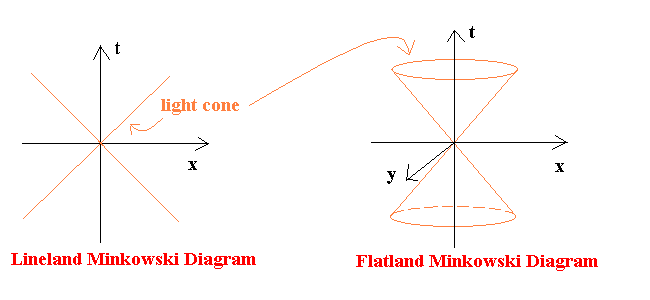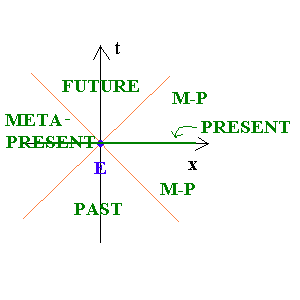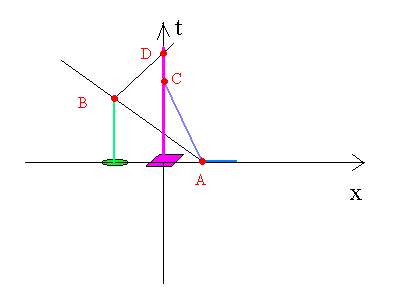# VISUALIZATION: Minkowski Diagrams

One frequently used method of visualizing spacetime is the Minkowski Diagram. Such diagrams are a subset of the general spacetime diagrams presented earlier. The defining feature of a Minkowski diagram is that light rays are drawn at a 45 degree angle to the line or plane respresenting space. This means that if the time axis is measured in seconds, then the space axes are measured in light-seconds (the distance light can travel in one second).

The two most common types of Minkowski Diagrams are "Lineland Minkowski Diagrams" and "Flatland Minkowski Diagrams". In a Lineland Minkowski Diagram, there is one axis for space (a line), and one axis for time. Thus, a Lineland Minkowski Diagram is a plane, with light rays traveling at 45 and 135 degree angles to the space axis (these rays make up a two dimensional light cone). In a Flatland Minkowski Diagram, there are two axes for space (a plane), and one axis for time. Hence, a Flatland Minkowski Diagram is a 3-Space, with light cones as in the diagram below.Figure 1: Minkowski Diagrams

An event (a particular place at a particular time) is represented by a point on the Minkowski Diagram. If light cones are drawn in the positive and negative time directions from a certain event (E), spacetime is separated into three distinct regions: "future", "past", and "meta-present". The future is the locus of all events (that have not yet happened) that E can (and does) affect. The reason that E cannot affect anything outside of it's future light cone is because, in order to do so, it would have to send some sort of message to the desired location faster than the speed of light. According to modern theory, this is impossible, for the speed of light is believed to be the greatest possible speed.

The past is the locus of events that contributed to E state. Anything that happened before E and is not in the past light cone could not have affected E (because its future light cone does not encompass E). Of note is the fact that any event can eventually become a part of another being's past.Figure 2: Regions of the Minkowski Diagram

The meta-present is a mysterious thing. One piece of the meta-present is the present, which are all of the events that lie on a line (or plane) of simultaneity with E--things that are actually happening at the same time. The author refers to the space between past and future as the "meta-present", because either a) the events have happened, and there is no way for someone at E to know about it or b) the events has not happened, but there is nothing that a person at E can do about it. For all intensive purposes, this is a good working definition of a "kind" of present.

As before, world lines can be drawn in Minkowski Diagrams. These represent a being's travel through spacetime, and are lines (or curves) for n-dimensional Minkowski Diagrams.

One example of the use of Minkowski Daigrams is as follows (refer to Figure 3): A Square conveniently rests at the origin of spacetime. A woman starts charging at him (A), with her sharp point threatening A Square. A high priest sees the woman attacking A Square (B), and sends him a warning signal of some kind (B). However, A Square is doomed. Even though the priest sent a warning before the woman gets to A Square, A Square is impaled (C).

Let's go to the video tape:Figure 3: A Square Having a Bad Day

B (the priest receiving the message) can do nothing about A Square's dilemma because C (A Square's death) is not in B's future light cone, and B is not in C's past light cone. B's warning eventually crosses A Square's world line (at D), but A Square is dead by this time. For more far-out interpretations of spacetime, see Conic Sections of Minkowski Diagram Light Cones.